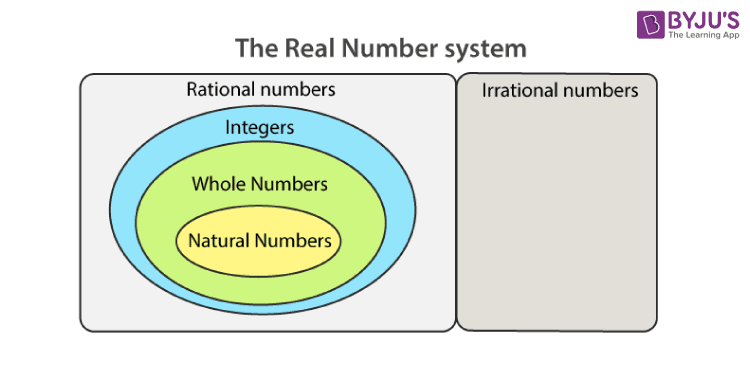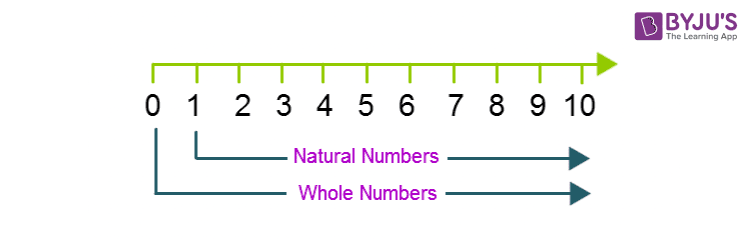# Whole Numbers

The whole numbers are the part of the number system which includes all the positive integers from 0 to infinity. These numbers exist in the number line. Hence, they are all real numbers. We can say, all the whole numbers are real numbers, but not all the real numbers are whole numbers. Thus, we can define whole numbers as the set of natural numbers and 0. Integers are the set of whole numbers and negative of natural numbers. Hence, integers include both positive and negative numbers including 0. Real numbers are the set of all these types of numbers, i.e., natural numbers, whole numbers, integers and fractions.

The complete set of natural numbers along with ‘0’ are called whole numbers. The examples are: 0, 11, 25, 36, 999, 1200, etc.

## Whole Numbers Definition

The whole numbers are the numbers without fractions and it is a collection of positive integers and zero. It is represented by the symbol “W” and the set of numbers are {0, 1, 2, 3, 4, 5, 6, 7, 8, 9,……………}. Zero as a whole represents nothing or a null value.

 Whole Numbers: W = {0, 1, 2, 3, 4, 5, 6, 7, 8, 9, 10……} Natural Numbers: N = {1, 2, 3, 4, 5, 6, 7, 8, 9,…} Integers: Z = {….-9, -8, -7, -6, -5, -4, -3, -2, -1, 0, 1, 2, 3, 4, 5, 6, 7, 8, 9,…} Counting Numbers: {1, 2, 3, 4, 5, 6, 7,….}

These numbers are positive integers including zero and do not include fractional or decimal parts (3/4, 2.2 and 5.3 are not whole numbers). Also, arithmetic operations such as addition, subtraction, multiplication and division are possible on whole numbers.

### Symbol

The symbol to represent whole numbers is the alphabet ‘W’ in capital letters.

W = {0, 1, 2, 3, 4, 5, 6, 7, 8, 9, 10,…}

Thus, the whole numbers list includes 0, 1, 2, 3, 4, 5, 6, 7, 8, 9, 10, 11, 12, ….

Facts:

 All the natural numbers are whole numbers All counting numbers are whole numbers All positive integers including zero are whole numbers All whole numbers are real numbers

If you still have doubt, What is a whole number in maths? A more comprehensive understanding of the whole numbers can be obtained from the following chart:## Whole Numbers Properties

The properties of whole numbers are based on arithmetic operations such as addition, subtraction, division and multiplication. Two whole numbers if added or multiplied will give a whole number itself. Subtraction of two whole numbers may not result in whole numbers, i.e. it can be an integer too. Also, the division of two whole numbers results in getting a fraction in some cases. Now, let us see some more properties of whole numbers and their proofs with the help of examples here.

### Closure Property

They can be closed under addition and multiplication, i.e., if x and y are two whole numbers then x. y or x + y is also a whole number.

Example:

5 and 8 are whole numbers.

5 + 8 = 13; a whole number

5 × 8 = 40; a whole number

Therefore, the whole numbers are closed under addition and multiplication.

### Commutative Property of Addition and Multiplication

The sum and product of two whole numbers will be the same whatever the order they are added or multiplied in, i.e., if x and y are two whole numbers, then x + y = y + x and x . y = y . x

Example:

Consider two whole numbers 3 and 7.

3 + 7 = 10

7 + 3 = 10

Thus, 3 + 7 = 7 + 3 .

Also,

3 × 7 = 21

7 × 3 = 21

Thus, 3 × 7 = 7 × 3

Therefore, the whole numbers are commutative under addition and multiplication.

When a whole number is added to 0, its value remains unchanged, i.e., if x is a whole number then x + 0 = 0 + x = x

Example:

Consider two whole numbers 0 and 11.

0 + 11 = 11

11 + 0 = 11

Here, 0 + 11 = 11 + 0 = 11

Therefore, 0 is called the additive identity of whole numbers.

### Multiplicative identity

When a whole number is multiplied by 1, its value remains unchanged, i.e., if x is a whole number then x.1 = x = 1.x

Example:

Consider two whole numbers 1 and 15.

1 × 15 = 15

15 × 1 = 15

Here, 1 × 15 = 15 = 15 × 1

Therefore, 1 is the multiplicative identity of whole numbers.

### Associative Property

When whole numbers are being added or multiplied as a set, they can be grouped in any order, and the result will be the same, i.e. if x, y and z are whole numbers then x + (y + z) = (x + y) + z and x. (y.z)=(x.y).z

Example:

Consider three whole numbers 2, 3, and 4.

2 + (3 + 4) = 2 + 7 = 9

(2 + 3) + 4 = 5 + 4 = 9

Thus, 2 + (3 + 4) = (2 + 3) + 4

2 × (3 × 4) = 2 × 12 = 24

(2 × 3) × 4 = 6 × 4 = 24

Here, 2 × (3 × 4) = (2 × 3) × 4

Therefore, the whole numbers are associative under addition and multiplication.

### Distributive Property

If x, y and z are three whole numbers, the distributive property of multiplication over addition is x. (y + z) = (x.y) + (x.z), similarly, the distributive property of multiplication over subtraction is x. (y – z) = (x.y) – (x.z)

Example:

Let us consider three whole numbers 9, 11 and 6.

9 × (11 + 6) = 9 × 17 = 153

(9 × 11) + (9 × 6) = 99 + 54 = 153

Here, 9 × (11 + 6) = (9 × 11) + (9 × 6)

Also,

9 × (11 – 6) = 9 × 5 = 45

(9 × 11) – (9 × 6) = 99 – 54 = 45

So, 9 × (11 – 6) = (9 × 11) – (9 × 6)

Hence, verified the distributive property of whole numbers.

### Multiplication by zero

When a whole number is multiplied to 0, the result is always 0, i.e., x.0 = 0.x = 0

Example:

0 × 12 = 0

12 × 0 = 0

Here, 0 × 12 = 12 × 0 = 0

Thus, for any whole number multiplied by 0, the result is always 0.

### Division by zero

The division of a whole number by o is not defined, i.e., if x is a whole number then x/0 is not defined.

Also, check: Whole number calculator

## Difference Between Whole Numbers and Natural Numbers

Difference Between Whole Numbers & Natural Numbers

Whole Numbers Natural Numbers
Whole Numbers: {0, 1, 2, 3, 4, 5, 6,…..} Natural Numbers: {1, 2, 3, 4, 5, 6,……}
Counting starts from 0 Counting starts from 1
All whole numbers are not natural numbers All Natural numbers are whole numbers

The below figure will help us to understand the difference between the whole number and natural numbers :### Can Whole Numbers be negative?

The whole number can’t be negative!

As per definition: {0, 1, 2, 3, 4, 5, 6, 7,……till positive infinity} are whole numbers. There is no place for negative numbers.

### Is 0 a whole number?

Whole numbers are the set of all the natural numbers including zero. So yes, 0 (zero) is not only a whole number but the first whole number.

## Solved Examples

Example 1: Are 100, 227, 198, 4321 whole numbers?

Solution: Yes. 100, 227, 198, and 4321 are all whole numbers.

Example 2: Solve 10 × (5 + 10) using the distributive property.

Solution:  Distributive property of multiplication over the addition of whole numbers is:

x × (y + z) = (x × y) + (x × z)

10 × (5 + 10) = (10 × 5) + (10 × 10)

= 50 + 100

= 150

Therefore, 10 × (5 + 10) = 150

However, we can show several examples of whole numbers using the properties of the whole numbers.

### Practice Problems

1. Write whole numbers between 12 and 25.
2. What is the additive inverse of the whole number 98?
3. How many whole numbers are there between -1 and 14?

To learn more concepts like natural numbers, and real numbers in a more engaging way, register at BYJU’S. Also, watch interesting videos on various maths topics by downloading BYJU’S– The Learning App from Google Play Store or the app store.

## Video lesson## Frequently Asked Questions on Whole Numbers

Q1

### What are whole numbers?

The whole numbers are defined as positive integers including zero. The whole number does not contain any decimal or fractional part. It means that it represents the entire thing without pieces. The set of whole numbers is mathematically represented as:
W = (0, 1, 2, 3, 4, 5,……}

Q2

### Can whole numbers be negative?

No, the whole numbers cannot be negative. The whole numbers start from 0, 1, 2, 3, … and so on. All the natural numbers are considered as whole numbers, but all the whole numbers are not natural numbers. Thus, the negative numbers are not considered as whole numbers.

Q3

### What are the properties of whole numbers?

The properties of whole numbers are:
Whole numbers are closed under addition and multiplication
The addition and multiplication of whole numbers is commutative
The addition and multiplication of whole numbers is associative
It obeys the distributive property of multiplication over addition
The additive identity of whole numbers is 0
The multiplicative identity of whole numbers is 1

Q4

### Is 10 a whole number?

10 is a whole as well as a natural number. It is written as Ten in words. Although -10 also represents a whole and not a fraction.
Q5

### Which numbers are not whole numbers?

The numbers which do not exist between 0 and infinity are not whole numbers. Negative integers, fractions or rational numbers are not whole numbers. Examples are -1, -5, ½, 9/4, pi, etc. are not whole numbers.
Q6

### Are all whole numbers real numbers?

Real numbers are those numbers that include rational numbers, integers, whole numbers and natural numbers. All whole numbers are real numbers but not all real numbers are whole.
Q7

### Are all natural numbers, whole numbers?

Natural numbers are those which start from 1 and end at infinity, whereas whole numbers start from 0 and end at infinity. All the natural numbers are whole numbers but not all whole numbers are natural.
Q8

### Are natural numbers and counting numbers the same?

Natural numbers are the numbers starting from 1 and extend up to infinity. Counting numbers are used to count the objects or people or anything which is countable. Hence, we always start counting from 1.
Test your knowledge on Whole Numbers

1. Explanation is given very beautifully

2. Hello
Good afternoon
Respected Mam / Sir
I want some questions and their full solutions with answer of Closure, Commutative, Associative and Distributive property so can l have the questions now
I am Prachi from class 6th
I am also using byju’s and l have purchased pack also of class 5th and 6th now in this year l came in 6th